﻿ 双层神经元网络中子网络的时空斑图研究 Spatio-Temporal Pattern in a Subnetwork of a Bi-Layer Neuronal Network

Biophysics
Vol.06 No.03(2018), Article ID:26562,7 pages
10.12677/BIPHY.2018.63005

Spatio-Temporal Pattern in a Subnetwork of a Bi-Layer Neuronal Network

Yanhong Zheng

College of Mathematics and Informatics & FJKLMAA, Fujian Normal University, Fuzhou Fujian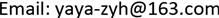Received: Jul. 31st, 2018; accepted: Aug. 15th, 2018; published: Aug. 23rd, 2018ABSTRACT

The firing behaviour and spatio-temporal pattern of neurons in the ring subnetwork of a bi-layer neuronal network coupled by a ring network and a grid network are studied in this paper. For the ring subnetwork, the firing behaviour and the spatio-temporal pattern of neurons are mainly influenced by the interlayer coupling strength. The grid-layer network will influence the firing behaviour of the neurons in ring-layer network with the interlayer coupling strength increasing.

Keywords:Bi-Layer Neuronal Network, Firing Behaviour, Spatio-Temporal PatternCopyright © 2018 by author and Hans Publishers Inc.1. 引言

2. 噪声环境下的环–格双层耦合网络模型

Morris-Lecar神经元模型是描述北极鹅肌肉纤维的电活动的一个神经元模型。这个模型能模拟现实神经元的激发和恢复的特性。模型包含了一个产生快的动作电位的一个Ca2+流和一个K+流。与此同时，为了维持休止态处的恒定电位，还考虑了一个漏电流。二维的ML神经元模型由下面的微分方程给出：

$\left\{\begin{array}{l}C\frac{\text{d}V}{\text{d}t}=-{g}_{L}\left(V-{V}_{L}\right)-{g}_{Ca}{m}_{\infty }\left(V\right)\left(V-{V}_{Ca}\right)-{g}_{K}n\left(V-{V}_{K}\right)+I,\\ \frac{\text{d}n}{\text{d}t}={\lambda }_{n}\left(V\right)\left({n}_{\infty }\left(V\right)-n\right),\end{array}$ (1)

${m}_{\infty }\left(V\right)=\frac{1}{2}\left\{1+\mathrm{tanh}\left[\left(V-{V}_{1}\right)/{V}_{2}\right]\right\},$

${n}_{\infty }\left(V\right)=\frac{1}{2}\left\{1+\mathrm{tanh}\left[\left(V-{V}_{3}\right)/{V}_{4}\right]\right\},$

${\lambda }_{n}\left(V\right)={\stackrel{¯}{\lambda }}_{n}\mathrm{cosh}\left[\left(V-{V}_{3}\right)/\left(2{V}_{4}\right)\right],$

$\left\{\begin{array}{l}C\frac{\text{d}{P}_{i}}{\text{d}t}=-{g}_{Ca}^{1}{m}_{\infty }\left({P}_{i}\right)\left({P}_{i}-{V}_{Ca}\right)-{g}_{K}{Q}_{i}\left({P}_{i}-{V}_{K}\right)-{g}_{L}\left({P}_{i}-{V}_{L}\right)+{I}_{1}\\ \text{}+{g}_{1}\left({P}_{i-1}+{P}_{i+1}-2{P}_{i}\right)+{g}_{3}{\left({V}_{i,i}-P\right)}_{i}+D{\xi }_{i},\\ \frac{\text{d}{Q}_{i}}{\text{d}t}={\lambda }_{n}\left({P}_{i}\right)\left({n}_{\infty }{\left(P\right)}_{i}-{Q}_{i}\right),\text{}i=1,\cdots ,N.\end{array}$ (2)

${m}_{\infty }\left({P}_{i}\right)=\frac{1}{2}\left\{1+\mathrm{tanh}\left[\left({P}_{i}-{V}_{1}\right)/{V}_{2}\right]\right\},$

${n}_{\infty }\left({P}_{i}\right)=\frac{1}{2}\left\{1+\mathrm{tanh}\left[\left({P}_{i}-{V}_{3}\right)/{V}_{4}\right]\right\},$

${\lambda }_{n}\left({P}_{i}\right)=\varphi \mathrm{cosh}\left[\left({P}_{i}-{V}_{3}\right)/\left(2{V}_{4}\right)\right],$

$\left\{\begin{array}{l}C\frac{\text{d}{V}_{i,j}}{\text{d}t}=-{g}_{Ca}^{*}{m}_{\infty }^{*}\left({V}_{i,j}\right)\left({V}_{i,j}-{V}_{Ca}\right)-{g}_{K}{W}_{i,j}\left({V}_{i,j}-{V}_{K}\right)-{g}_{L}\left({V}_{i,j}-{V}_{L}\right)+{I}_{2}\\ \text{}+{g}_{2}\left({V}_{i-1,j}+{V}_{i+1,j}+{V}_{i,j-1}+{V}_{i,j+1}-4{V}_{i,j}\right)+{g}_{3}\left({P}_{i}-{V}_{i,i}\right)+D{\xi }_{i,j},\\ \frac{\text{d}{W}_{i,j}}{\text{d}t}={\lambda }_{n}^{*}\left({V}_{i,j}\right)\left({n}_{\infty }^{*}\left({V}_{i,j}\right)-{W}_{i,j}\right),\text{}i,j=1,\cdots ,N.\end{array}$ (3)

${m}_{\infty }^{*}\left({V}_{i,j}\right)=\frac{1}{2}\left\{1+\mathrm{tanh}\left[\left({V}_{i,j}-{V}_{1}\right)/{V}_{2}\right]\right\},$

${n}_{\infty }^{*}\left({V}_{i,j}\right)=\frac{1}{2}\left\{1+\mathrm{tanh}\left[\left({V}_{i,j}-{V}_{3}^{*}\right)/{V}_{4}^{*}\right]\right\},$

${\lambda }_{n}^{*}\left({V}_{i,j}\right)={\varphi }_{2}\mathrm{cosh}\left[\left({V}_{i,j}-{V}_{3}^{*}\right)/\left(2{V}_{4}^{*}\right)\right],$

3. 环式子网络A的神经元放电行为和时空斑图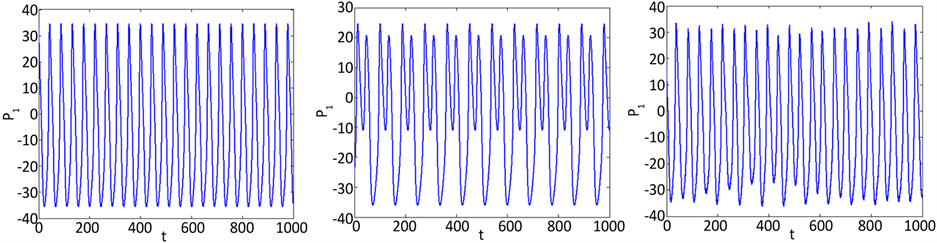(a) (b) (c)

Figure 1. Time series of the first neuron in ring-layer A: (a) D = 0, g3 = 0; (b) D = 0, g3 = 2; (c) D = 0.3, g3 = 0.5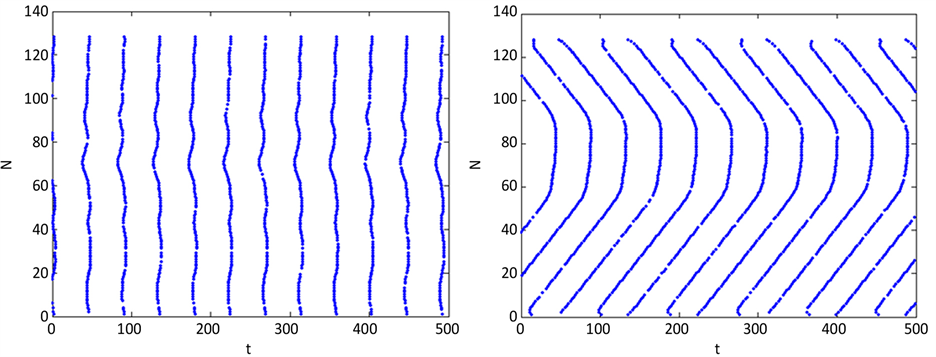(a) (b)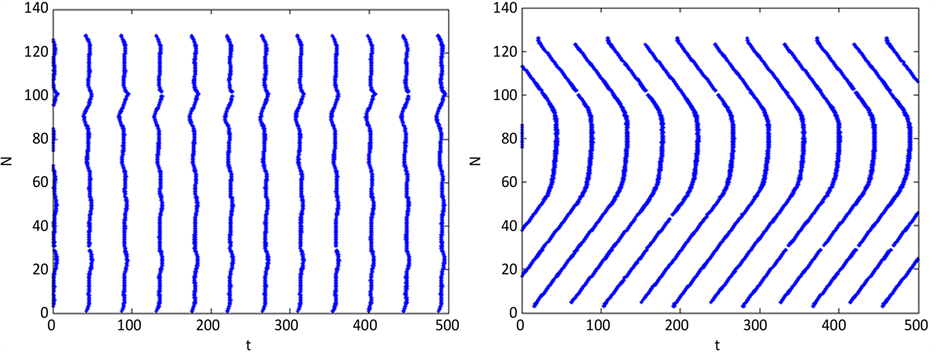(c) (d)

Figure 2. Spatio-temporal firing patterns of subnetwork A in a raster plot: (a) D = 0, g3 = 0; (b) D = 0, g3 = 2; (c) D = 0.1, g3 = 0.08; (d) D = 0.1, g3 = 2

${c}_{i}\left({\tau }_{d}\right)=\frac{\left[{x}_{i}\left(t\right)-〈{x}_{i}\left(t\right)〉\right]\left[{x}_{i}\left(t+{\tau }_{d}\right)-〈{x}_{i}\left(t\right)〉\right]}{〈{\left[{x}_{i}\left(t\right)-〈{x}_{i}\left(t\right)〉\right]}^{2}〉}$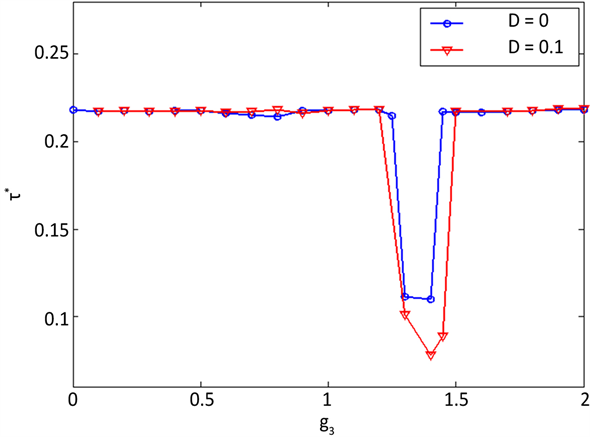Figure 3. Variation of the order parameter ${\tau }^{*}$ with respect to the interlayer coupling strength g3 for D = 0 and D = 0.1, respectively

4. 结论

Spatio-Temporal Pattern in a Subnetwork of a Bi-Layer Neuronal Network[J]. 生物物理学, 2018, 06(03): 50-56. https://doi.org/10.12677/BIPHY.2018.63005

1. 1. Morris, C. and Lecar, H. (1981) Voltage Oscillations in the Bar-nacle Giant Muscle Fiber. Biophysical Journal, 35, 193-213. https://doi.org/10.1016/S0006-3495(81)84782-0

2. 2. Pikovsky, A.S. and Kurths, J. (1997) Coherence Resonance in a Noise-Driven Excitable System. Physical Review Letters, 78, 775-778. https://doi.org/10.1103/PhysRevLett.78.775

3. 3. 方小玲, 于洪洁. 复杂脑网络研究进展[J]. 力学进展, 2007, 37(4): 611-613.

4. 4. Sun, X.J., Lei, J.Z., Kurths, J., Lv, S.J. and Lu, Q.S. (2011) Burst Synchronization Transition in a Neuronal Network of Subnetworks. Chaos, 21, Article ID: 016110. https://doi.org/10.1063/1.3559136

5. 5. Lameu, E.L., Batista, C.A.S., Batista, A.M., Iarosz, K., Viana, R.L., Lopes, S.R. and Kurths, J. (2012) Suppression of Bursting Synchronization in Clustered Scale-Free (Rich-Club) Neuronal Networks. Chaos, 22, Article ID: 043149. https://doi.org/10.1063/1.4772998

6. 6. Batista, C.A.S., Lameu, E.L., Batista, A.M., Lopes, S.R., Pereira, T., Za-mora-López, G., et al. (2012) Phase Synchronization of Bursting Neurons in Clustered Small-World Networks. Physical Review E, 86, Article ID: 016211. https://doi.org/10.1103/PhysRevE.86.016211

7. 7. Zheng, Y.H. and Wang, H.X. (2015) Chaotic Burst Synchro-nization in a Two-Small-World-Layer Neuronal Network. International Journal of Modern Physics C, 26, Article ID: 1550051. https://doi.org/10.1142/S0129183115500515

8. 8. Nicholls, J.G.., et al. 神经生物学——从神经元到脑[M]. 北京: 科学出版社, 2003.

9. 9. Sun, X.J. and Lu, Q.S. (2009) Pattern Synchronization in a Two-Layer Neu-ronal Network. Chinese Physics Letters, 26, Article ID: 060507.

10. 10. Yu, H.T, Wang, J., Liu, C., Deng, B. and Wei, X.L. (2011) Vibrational Resonance in Excitable Neuronal Systems. Chaos, 21, Article ID: 043101. https://doi.org/10.1063/1.3644390

11. 11. Zhou, C.S., Zemanová, L., Zamora-López, G., et al. (2007) Struc-ture-Function Relationship in Complex Brain Networks Expressed by Hierarchical Synchronization. New Journal of Physics, 9, 178. https://doi.org/10.1088/1367-2630/9/6/178